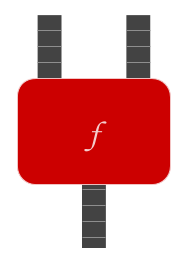# Function: Physical metaphor

Many functions can be visualized as physical mechanisms of wheels and gears, that take their inputs on conveyor belts, manipulate them (using mechanical sensors and tools), and produce an output which is placed on an outgoing conveyor belt.For example, we can visualize the function $$+$$ as a function that takes two stacks of poker chips in as input (one on the left conveyor belt and one on the right conveyor belt) and produces its output by dumping both stacks onto the output belt. We can visualize the function $$\times$$ as a function that also gets a stack of poker chips on each input belt, and which puts a copy of the left stack onto the output tape for each poker chip in the right stack.

All computable functions can (in principle) be implemented by a physical system of wheels and gears (or circuits and wires, etc.), and it is conjectured that the computable functions are the only functions that can be implemented by physical systems. (This conjecture is known as the Church-Turing thesis.)

Parents: